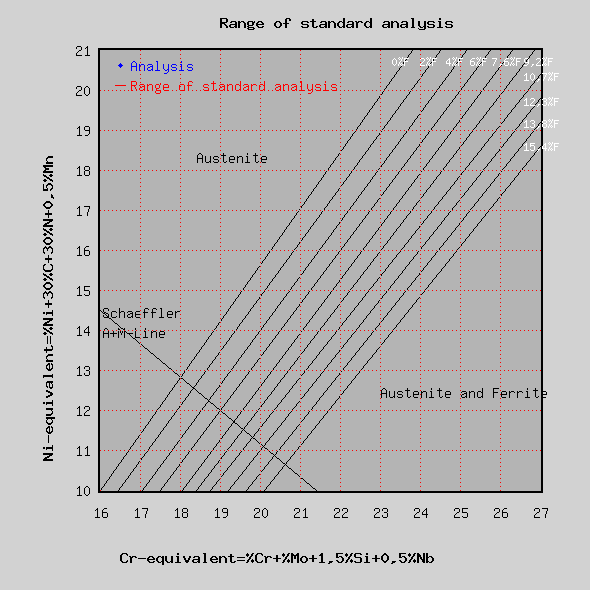# De Long diagram for range of standard analysis

The nickel and the chromium equivalent provide information about the amount of the various structurs in stainless steels. Nickel and chromium are contained in such steels in considerable amounts. Nickel creates austenite and chromium creates ferrite. By entering the Ni-equivalent over the Cr-equivalent for stainless steel into a diagram according to De Long one is able to find the content of austenite and ferrite in the resulting microstructure. In opposite to the Schaeffler-diagram nitrogen is taken into consideration with the nickel equivalent. This diagram permits a preciser evaluation of the ferrite content.

Calculation

After entering the range of the standard analysis and the actual analysis the Ni-equivalent and the Cr-equivalent are calculated and shown in a diagram.

The sample data can be overridden and the red backgrounded fields show the results.

 Composition Analysis Minimum Maximum Carbon C [%] Silicon Si [%] Manganese Mn [%] Chromium Cr [%] Molybdenum Mo [%] Nickel Ni [%] Niobium Nb [%] Nitrogen  N [%] Chromium equivalent  [%] Nickel equivalent  [%]